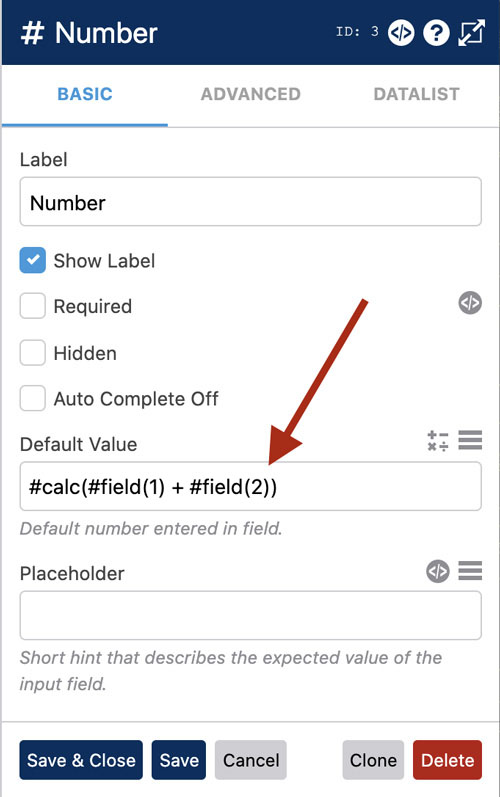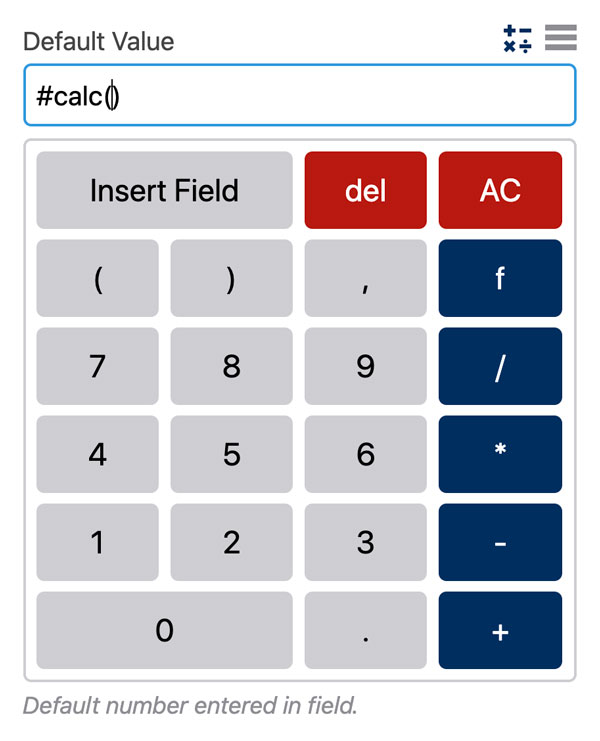# Calculated FieldsPRO

The `#calc` calculated fields function in WS Form PRO makes it easy to create calculate field values that are updated in realtime. It can handle everything from simple addition to more complex trigonometric functions.

## How To Create A Calculated Field

Common field types you might use a calculated field in include:

You can put calculated values in the following settings of a field:

• Label
• Default value
• Content (Text Editor / HTML fields)
• Placeholder
• Help
• Minimum
• Maximum

To create a calculated field value for the default value of a field, click the field settings icon and then enter a `#calc(...)` variable in the Default Value setting.Anything within the brackets will be calculated in realtime on your form and used as the value of your field.

You can create calculations such as:

`#calc(1 + 2)`

You can include values from other fields on your form, for example, multiplying field ID 123 value by 5:

`#calc(#field(123) * 5)`

You can use WS Form math variables (listed at the end of this document) to perform more complex calculations, for example, rounding the previous example to two decimal places:

`#calc(#round(#field(123) * 5, 2))`

You could even create a calculated field that works out the sum of all values of a field in a repeatable section. The syntax is easy:

`#calc(#field(123))`

… where field ID 123 is a field in a repeatable section. WS Form PRO automatically sums the values together.  The `#section_row_count(456)` function (where 456 is the section ID) can be used to calculate the average value of those values:

`#calc(#field(123) / #section_row_count(456))`

There is an easier way of creating these calculations … the calculator!

### Calculator

WS Form PRO includes a calculator feature that helps creating calculated values for fields.

1. Click the field settings icon.
2. Click the calculator icon. On most fields this is found on the Default Value setting.

The calculator will then appear directly below:The cursor will automatically position itself within the #calc() function. In addition to the normal numeric and arithmetic buttons, the calculator has the following additional buttons:

Insert Field – Click this to insert a #field() variable into your calculated field. It lists all of the fields on your form that are compatible with the calculated field feature.

del – Deletes a character

AC (All Clear) – Deletes the entire calculation

f – Inserts a math variable into your calculation such as `#pi`, `#cos(...)` or `#sin(...)` (See below)

Note that whilst the calculator will do its best to maintain a suitable cursor position, you can always click on the default value itself to reposition the cursor.

### Source Field Rules

If a field used in a calculation is an e-commerce type (e.g. price, price select etc), the input value is assumed to be a currency and WS Form will therefore correctly interpret the price according to your currency symbol, decimal and thousand separator settings and will convert that to a floating point number.

If a field used in a calculation is not an e-commerce type (e.g. number, text, select etc), the input value should follow standard conventions used for evaluating numbers in JavaScript, i.e.

• Period for decimal separator
• No thousand separators

For example: `123.45`

### Display Calculations as Prices

If you wish to show a calculation as a price using your currency settings, you can use the `ecommerce_price` variable, for example:

`#ecommerce_price(#calc(#field(1) + #field(2)))`

### JavaScript Floating Point Rounding

WS Form calculations are assessed using standard JavaScript. In some cases this can result in floating point numbers such as:

`123.40000000000001` instead of `123.4`

This is actually perfectly normal in the computing world! If you’re interested here is an explanation.

In order to get a neater looking number, simply use the `#round` variable, for example:

`#round(#calc(#field(1) + #field(2)), 1)`

The last parameter is the number of decimal places you would like to use.

### Variables

In addition to `#field(...)`, the following variables can be used inside with `#calc(...)`.

#### Math

Absolute
`#abs(number)`
Returns the absolute value of a number.
Average
`#avg(number)`
Returns the average of all the input numbers.
Base to the Exponent Power
`#pow(base, exponent)`
Returns the base to the exponent power.
Ceiling
`#ceil(number)`
Rounds a number up to the next largest whole number.
Cosine
`#cos(radians)`
Returns the cosine of a radian number.
Euler's
`#exp(number)`
Returns E to the power of a number.
Floor
`#floor(number)`
Returns the largest integer value that is less than or equal to a number.
Logarithm
`#log(number)`
Returns the natural logarithm of a number.
Maximum
`#max(number)`
Returns the maxiumum value of the supplied numbers.
Minimum
`#min(number)`
Returns the lowest value of the supplied numbers.
Negative
`#negative(number)`
Returns 0 if positive, or original value if negative.
PI
`#pi`
Returns an approximate value of PI.
Positive
`#positive(number)`
Returns 0 if negative, or original value if positive.
Round
`#round(number, decimals)`
Returns the rounded value of a number.
Sine
`#sin(radians)`
Returns the sine of a radian number.
Square Root
`#sqrt(number)`
Returns the square root of the number.
Tangent
`#tan(radians)`
Returns the tangent of a radian number.

#### Number

Format Number
`#number_format(number, decimals, decimal_separator, thousand_separator)`
Returns a number with grouped thousands. Same as the PHP number_format function.

#### Random Numbers

Random Number
`#random_number(min, max)`
Outputs an integer between the specified minimum and maximum attributes. This function does not generate cryptographically secure values, and should not be used for cryptographic purposes.

#### Seconds

Seconds in a day
`#seconds_day`
Returns the number of seconds in a day.
Seconds in a minute
`#seconds_minute`
Returns the number of seconds in a minute.
Seconds in a week
`#seconds_week`
Returns the number of seconds in a week.
Seconds in a year
`#seconds_year`
Returns the number of seconds in a common year.
Seconds in an hour
`#seconds_hour`
Returns the number of seconds in an hour.
Seconds since Epoch
`#seconds_epoch`
Returns the number of seconds since the Unix Epoch (January 1 1970 00:00:00 GMT).
Seconds since Epoch at midnight
`#seconds_epoch_midnight`
Returns the number of seconds since the Unix Epoch (January 1 1970 00:00:00 GMT) to the closest previous midnight. This variable can only be used in client-side.